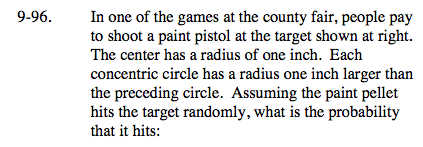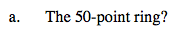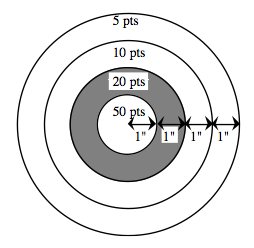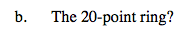### Home > A2C > Chapter Ch9 > Lesson 9.2.2 > Problem9-96

9-96.Area of a circle = πr2$P(50pt)=\frac{\text{area of success}}{\text{total area}}=\frac{\pi(1)^{2}}{\pi(4)^{2}}$

$\frac{1}{16}$$P(20pt)=\frac{\text{area of success}}{\text{total area}}=\frac{\pi(2)^{2}-\pi(1)^{2}}{\pi(4)^{2}}$